# Create Plot

Interactively create linear analysis response plots in the Live Editor

## Description

### Supported plots

The Create Plot Live Editor task supports the following linear analysis response plots:

## Open the Create Plot

To add the Create Plot task to a live script in the MATLAB Live Editor:

• On the Live Editor tab, click Task and select the Create Plot icon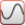.

• In a code block in the live script, type a relevant keyword, such as such as `bode`, `step`, `pzplot`, or `impulse`. Select Create Plot from the suggested command completions. When you add the task using this method, then MATLAB automatically selects the corresponding chart type in the Select visualization section of the task.

## Examples

expand all

Use the Create Plot task in the Live Editor to interactively create and visualize different linear analysis plots. You can also use an options object from your MATLAB® workspace to customize your plot. Open this example to see a preconfigured script containing the Create Plot task to create a Bode plot.

For this example, consider the following SISO state-space model:

`$A=\left[\begin{array}{cc}-1.5& -2\\ 1& 0\end{array}\right]\phantom{\rule{0.2777777777777778em}{0ex}}\phantom{\rule{0.2777777777777778em}{0ex}}\phantom{\rule{0.2777777777777778em}{0ex}}\phantom{\rule{0.2777777777777778em}{0ex}}B=\left[\begin{array}{c}0.5\\ 0\end{array}\right]\phantom{\rule{0.2777777777777778em}{0ex}}\phantom{\rule{0.2777777777777778em}{0ex}}\phantom{\rule{0.2777777777777778em}{0ex}}\phantom{\rule{0.2777777777777778em}{0ex}}C=\left[\begin{array}{cc}0& 1\end{array}\right]\phantom{\rule{0.2777777777777778em}{0ex}}\phantom{\rule{0.2777777777777778em}{0ex}}\phantom{\rule{0.2777777777777778em}{0ex}}\phantom{\rule{0.2777777777777778em}{0ex}}D=0$`

Create the SISO state-space model defined by the following state-space matrices:

```A = [-1.5,-2;1,0]; B = [0.5;0]; C = [0,1]; D = 0; sys = ss(A,B,C,D);```

To create a Bode plot of `sys`, open the Create Plot task in the Live Editor. On the Live Editor tab, select Task > Create Plot. In the task, select `Controls Toolbox Plots` from the Filter by Category dropdown menu. You can also directly search for the plot type by typing in the plot name in the Select visualization field.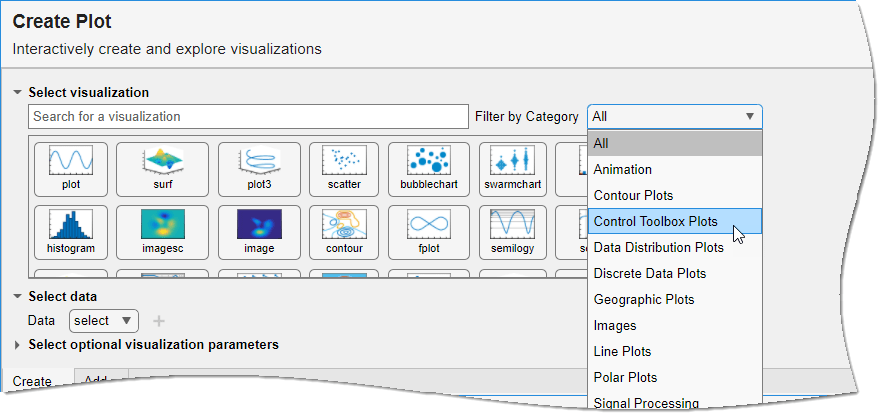Next, select bodeplot from the available plots. If you do not have a supported model type in your MATLAB workspace, then the respective plot type will not be available.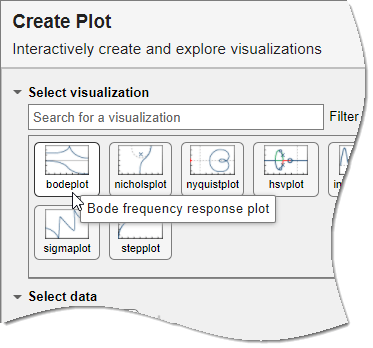Use the Dynamic System dropdown menu to pick the appropriate model from your MATLAB workspace. You can use the Frequencies dropdown menu to specify a vector of frequencies. You can also customize your plot by using a predefined options object. For more information, see `bodeplot`.

For this example, pick `sys` and leave the other options as `default`.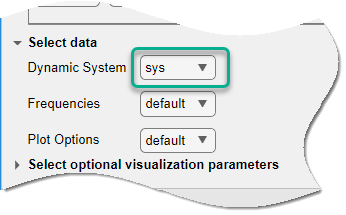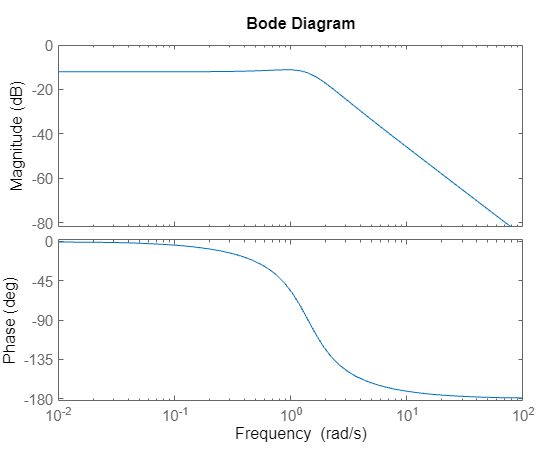You can also select the color, line style and marker symbol for the plot using the Select optional visualization parameters menu. You can click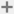to add more visualization parameters. For this example, change the line color to green.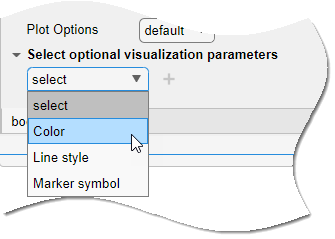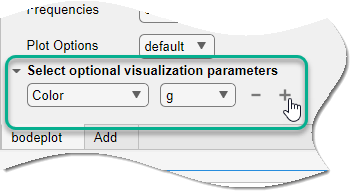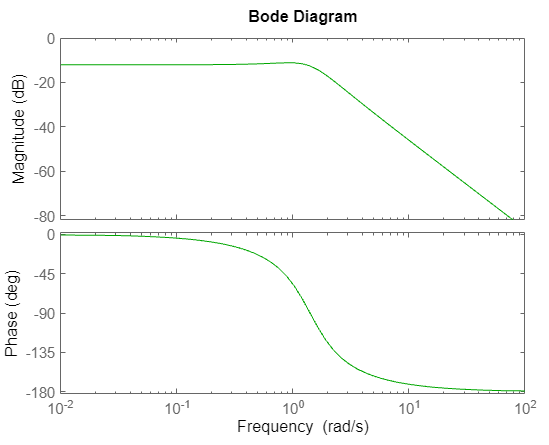To see the code that this task generates, expand the task display by clicking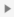at the bottom of the task parameter area.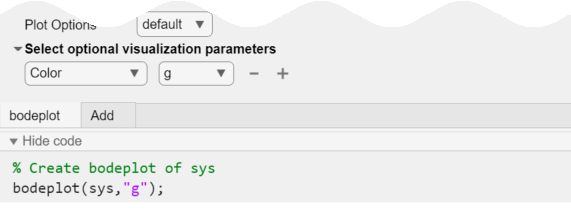You can also overlay mutliple visualizations on the same plot axis. For more information and examples, see the Create Plot task reference page.

## Parameters

Choose the dynamic system model or model array to create the linear analysis response plot. Dynamic systems that you can use include:

• Continuous-time or discrete-time numeric LTI models, such as `tf`, `zpk`, or `ss` models.

• Sparse state-space models, such as `sparss` or `mechss` models.

• Generalized or uncertain LTI models such as `genss` or `uss` (Robust Control Toolbox) models. (Using uncertain models requires Robust Control Toolbox™ software.)

• For tunable control design blocks, the task evaluates the model at its current value to plot the response data.

• For uncertain control design blocks, the task plots the nominal value and random samples of the model.

• Frequency-response data models such as `frd` models to plot the Bode, sigma, Nyquist or Nichols response. For such models, the task plots the response at frequencies defined in the model.

• Identified LTI models, such as `idtf` (System Identification Toolbox), `idss` (System Identification Toolbox), or `idproc` (System Identification Toolbox) models. (Using identified models requires System Identification Toolbox™ software.)

Note

• You can only use a single model to plot Hankel singular values. For all other response plots, the task plots the responses of all models in the array on the same axes when you have an array of dynamic system models.

Create and choose the correct options set based on the plot type as follows:

 Plot Type Options Object Creation Function `bodeplot` `BodeOptions` object `bodeoptions` `sigmaplot` `SigmaOptions` object `sigmaoptions` `nyquistplot` `NyquistOptions` object `nyquistoptions` `nicholsplot` `NicholsOptions` object `nicholsoptions` `stepplot` `TimeOptions` object `timeoptions` `impulseplot` `TimeOptions` object `timeoptions` `rlocusplot` `PZOptions` object `pzoptions` `pzplot` `PZOptions` object `pzoptions` `iopzplot` `PZOptions` object `pzoptions` `hsvplot` `HSVOptions` object `hsvoptions`

## Version History

Introduced in R2022b